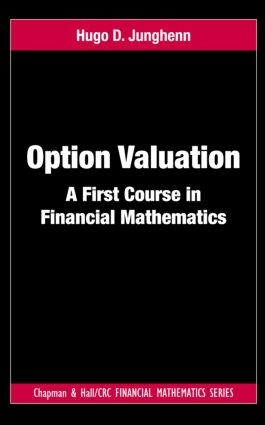# Option Valuation

## A First Course in Financial Mathematics, 1st Edition

Chapman and Hall/CRC

266 pages | 10 B/W Illus.

Hardback: 9781439889114
pub: 2011-11-23
SAVE ~\$14.59
\$72.95
\$58.36
x
eBook (VitalSource) : 9780429086236
pub: 2011-11-23
from \$36.48

FREE Standard Shipping!

### Description

Option Valuation: A First Course in Financial Mathematics provides a straightforward introduction to the mathematics and models used in the valuation of financial derivatives. It examines the principles of option pricing in detail via standard binomial and stochastic calculus models. Developing the requisite mathematical background as needed, the text presents an introduction to probability theory and stochastic calculus suitable for undergraduate students in mathematics, economics, and finance.

The first nine chapters of the book describe option valuation techniques in discrete time, focusing on the binomial model. The author shows how the binomial model offers a practical method for pricing options using relatively elementary mathematical tools. The binomial model also enables a clear, concrete exposition of fundamental principles of finance, such as arbitrage and hedging, without the distraction of complex mathematical constructs. The remaining chapters illustrate the theory in continuous time, with an emphasis on the more mathematically sophisticated Black-Scholes-Merton model.

Largely self-contained, this classroom-tested text offers a sound introduction to applied probability through a mathematical finance perspective. Numerous examples and exercises help students gain expertise with financial calculus methods and increase their general mathematical sophistication. The exercises range from routine applications to spreadsheet projects to the pricing of a variety of complex financial instruments. Hints and solutions to odd-numbered problems are given in an appendix and a full solutions manual is available for qualifying instructors.

### Reviews

"…a suitable text for an advanced undergraduate or graduate-level course in option valuation via the binomial model and the Black–Scholes–Merton model."

International Statistical Review, 2013

"The text provides an introduction to classical material of mathematical finance, i.e. the notions of arbitrage, replication, and option pricing in the context of the discrete-time Cox-Ross-Rubinstein and the continuous-time Black-Scholes model, respectively. The book sticks out by not assuming any background in stochastics. All necessary concepts of probability theory, martingales, and Itô calculus are provided …"

—Jan Kallsen, Zentralblatt MATH 1247

Interest and Present Value

Compound Interest

Annuities

Bonds

Rate of Return

Probability Spaces

Sample Spaces and Events

Discrete Probability Spaces

General Probability Spaces

Conditional Probability

Independence

Random Variables

Definition and General Properties

Discrete Random Variables

Continuous Random Variables

Joint Distributions

Independent Random Variables

Sums of Independent Random Variables

Options and Arbitrage

Arbitrage

Classification of Derivatives

Forwards

Currency Forwards

Futures

Options

Properties of Options

Dividend-Paying Stocks

Discrete-Time Portfolio Processes

Discrete-Time Stochastic Processes

Self-Financing Portfolios

Option Valuation by Portfolios

Expectation of a Random Variable

Discrete Case: Definition and Examples

Continuous Case: Definition and Examples

Properties of Expectation

Variance of a Random Variable

The Central Limit Theorem

The Binomial Model

Construction of the Binomial Model

Pricing a Claim in the Binomial Model

The Cox-Ross-Rubinstein Formula

Conditional Expectation and Discrete-Time Martingales

Definition of Conditional Expectation

Examples of Conditional Expectation

Properties of Conditional Expectation

Discrete-Time Martingales

The Binomial Model Revisited

Martingales in the Binomial Model

Change of Probability

American Claims in the Binomial Model

Stopping Times

Optimal Exercise of an American Claim

Dividends in the Binomial Model

The General Finite Market Model

Stochastic Calculus

Differential Equations

Continuous-Time Stochastic Processes

Brownian Motion

Variation of Brownian Paths

Riemann-Stieltjes Integrals

Stochastic Integrals

The Ito-Doeblin Formula

Stochastic Differential Equations

The Black-Scholes-Merton Model

The Stock Price SDE

Continuous-Time Portfolios

The Black-Scholes-Merton PDE

Properties of the BSM Call Function

Continuous-Time Martingales

Conditional Expectation

Martingales: Definition and Examples

Martingale Representation Theorem

Moment Generating Functions

Change of Probability and Girsanov’s Theorem

The BSM Model Revisited

Risk-Neutral Valuation of a Derivative

Proofs of the Valuation Formulas

Valuation under P

The Feynman-Kac Representation Theorem

Other Options

Currency Options

Forward Start Options

Chooser Options

Compound Options

Path-Dependent Derivatives

Quantos

Options on Dividend-Paying Stocks

American Claims in the BSM Model

Appendix A: Sets and Counting

Appendix B: Solution of the BSM PDE

Appendix C: Analytical Properties of the BSM Call Function

Appendix D: Hints and Solutions to Odd-Numbered Problems

Bibliography

Index

Exercises appear at the end of each chapter.

Hugo D. Junghenn is a professor of mathematics at the George Washington University. His research interests include functional analysis and semigroups.